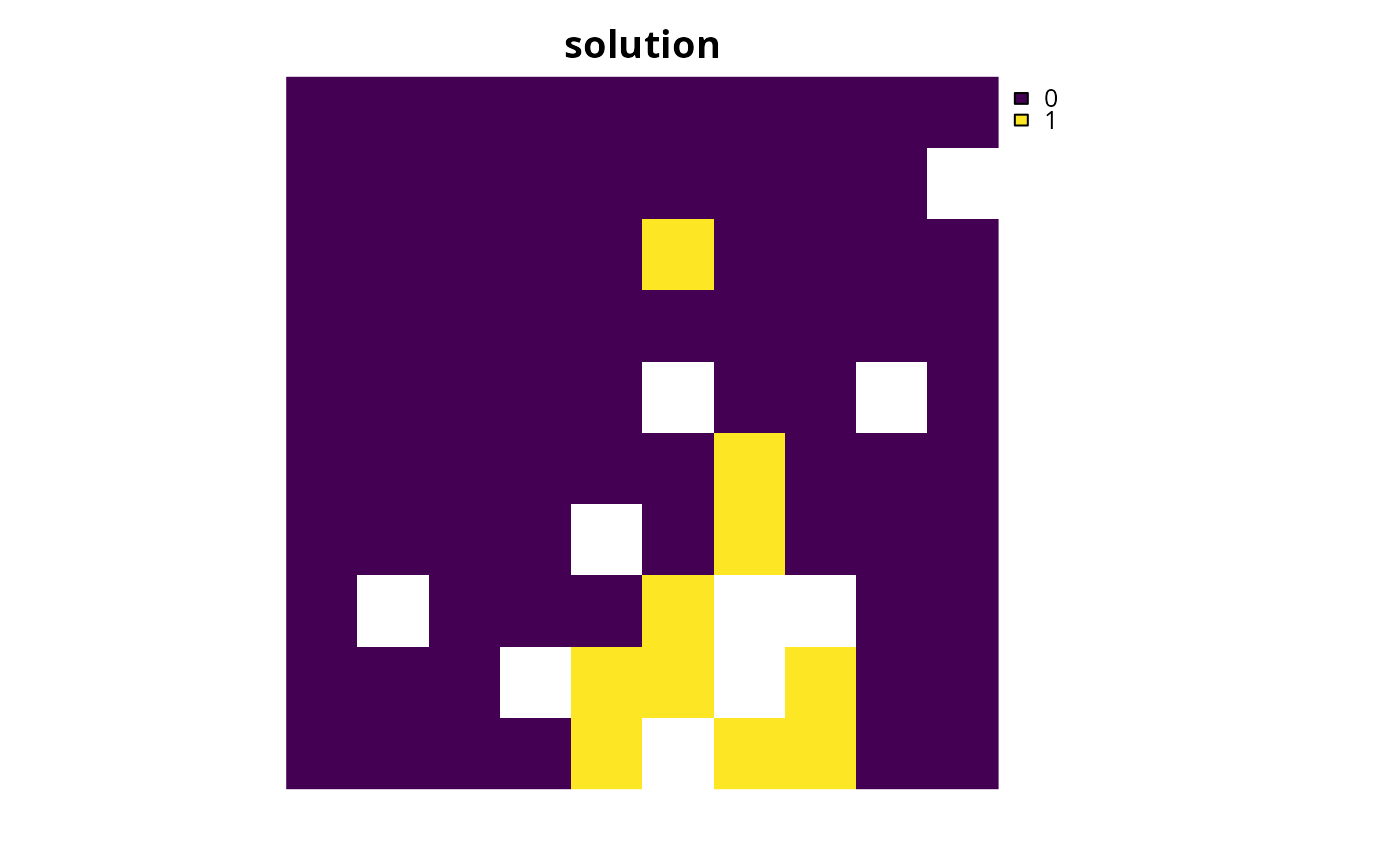Specify that the HiGHS software should be used to solve a conservation planning problem (Huangfu and Hall 2018). This function can also be used to customize the behavior of the solver. It requires the highs package to be installed.

add_highs_solver(
x,
gap = 0.1,
time_limit = .Machine$integer.max, presolve = TRUE, threads = 1, verbose = TRUE ) ## Arguments x problem() object. gap numeric gap to optimality. This gap is relative and expresses the acceptable deviance from the optimal objective. For example, a value of 0.01 will result in the solver stopping when it has found a solution within 1% of optimality. Additionally, a value of 0 will result in the solver stopping when it has found an optimal solution. The default value is 0.1 (i.e., 10% from optimality). time_limit numeric time limit (seconds) for generating solutions. The solver will return the current best solution when this time limit is exceeded. The default value is the largest integer value (i.e., .Machine$integer.max), effectively meaning that solver will keep running until a solution within the optimality gap is found.

presolve

logical attempt to simplify the problem before solving it? Defaults to TRUE.

integer number of threads to use for the optimization algorithm. The default value is 1.

verbose

logical should information be printed while solving optimization problems? Defaults to TRUE.

## Value

An updated problem() object with the solver added to it.

## Details

HiGHS is an open source optimization software. Although this solver can have comparable performance to the CBC solver (i.e., add_cbc_solver()) for particular problems and is generally faster than the SYMPHONY based solvers (i.e., add_rsymphony_solver(), add_lpsymphony_solver()), it can sometimes take much longer than the CBC solver for particular problems. This solver is recommended if the add_gurobi_solver(), add_cplex_solver(), add_cbc_solver() cannot be used.

Other solvers: add_cbc_solver(), add_cplex_solver(), add_default_solver(), add_gurobi_solver(), add_lsymphony_solver, add_rsymphony_solver()

## Examples

# \dontrun{
sim_pu_raster <- get_sim_pu_raster()
sim_features <- get_sim_features()

# create problem
p <-
problem(sim_pu_raster, sim_features) %>%